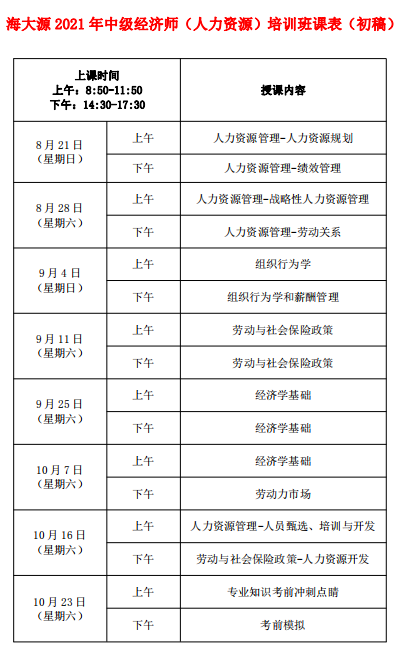66185800  66298600内容 单选题 多选题 总分 第一部分  经济学基础 15×1=15分 7×2=14分 29分 第二部分  财政 11×1=11分 6×2=12分 23分 第三部分  货币与金融 11×1=11分 6×2=12分 23分 第四部分  统计 11×1=11分 5×2=10分 21分 第五部分  会计 11×1=11分 5×2=10分 21分 第六部分  法律 11×1=11分 6×2=12分 23分 合计 70×1=70分 35×2=70分 140分1.2021年海南经济师中级职称考试培训招生简章
3.2021年海南中级经济师培训课程初稿

0898-66298600（海大源）

13016293330（敖主任）<--这段代码是专属于这个站点的：www.wuyer.net 此段代码添加前，请勿添加到其他站点，否则将无法正常使用。-->